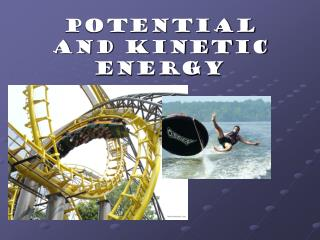DownloadDownload PresentationPotential and Kinetic Energy

# Potential and Kinetic Energy

Download Presentation## Potential and Kinetic Energy

- - - - - - - - - - - - - - - - - - - - - - - - - - - E N D - - - - - - - - - - - - - - - - - - - - - - - - - - -
##### Presentation Transcript

1. Potential and Kinetic Energy

2. Kinetic Energy Potential Energy Elastic Potential Energy Gravitation Potential Energy Chemical Potential Energy How is all energy divided? All Energy

3. What is Potential Energy? • Energy that is stored and waiting to be used later

4. What is Gravitational Potential Energy? • Potential energy due to an object’s position • P.E. = mass x height x gravity Don’t look down, Rover! Good boy!

5. What is Elastic Potential Energy? • Potential energy due compression or expansion of an elastic object. Notice the ball compressing and expanding

6. What is Chemical Potential Energy? • Potential energy stored within the chemical bonds of an object

7. What is Kinetic Energy? • Energy an object has due to its motion • K.E. = 1/2mv2

8. Kinetic Energy Depends on 2 factors If Dino and the car have the same velocity, which has the greater KE? If the trucks have the same mass, which one has greater KE? Mass and Velocity

9. Which factor has the greater impact on KE? Velocity has greater influence because it is SQUARED in the formula!! Think about the formula!!

10. Energy is Conserved!

11. The total amt of energy remains constant!! KE i + PE i = KE f + PE f

12. Mechanical Energy The total amount of KE and PE is referred to as the total MECHANICAL ENERGY of an object. TME = KE + PE

13. There are other forms of energy.